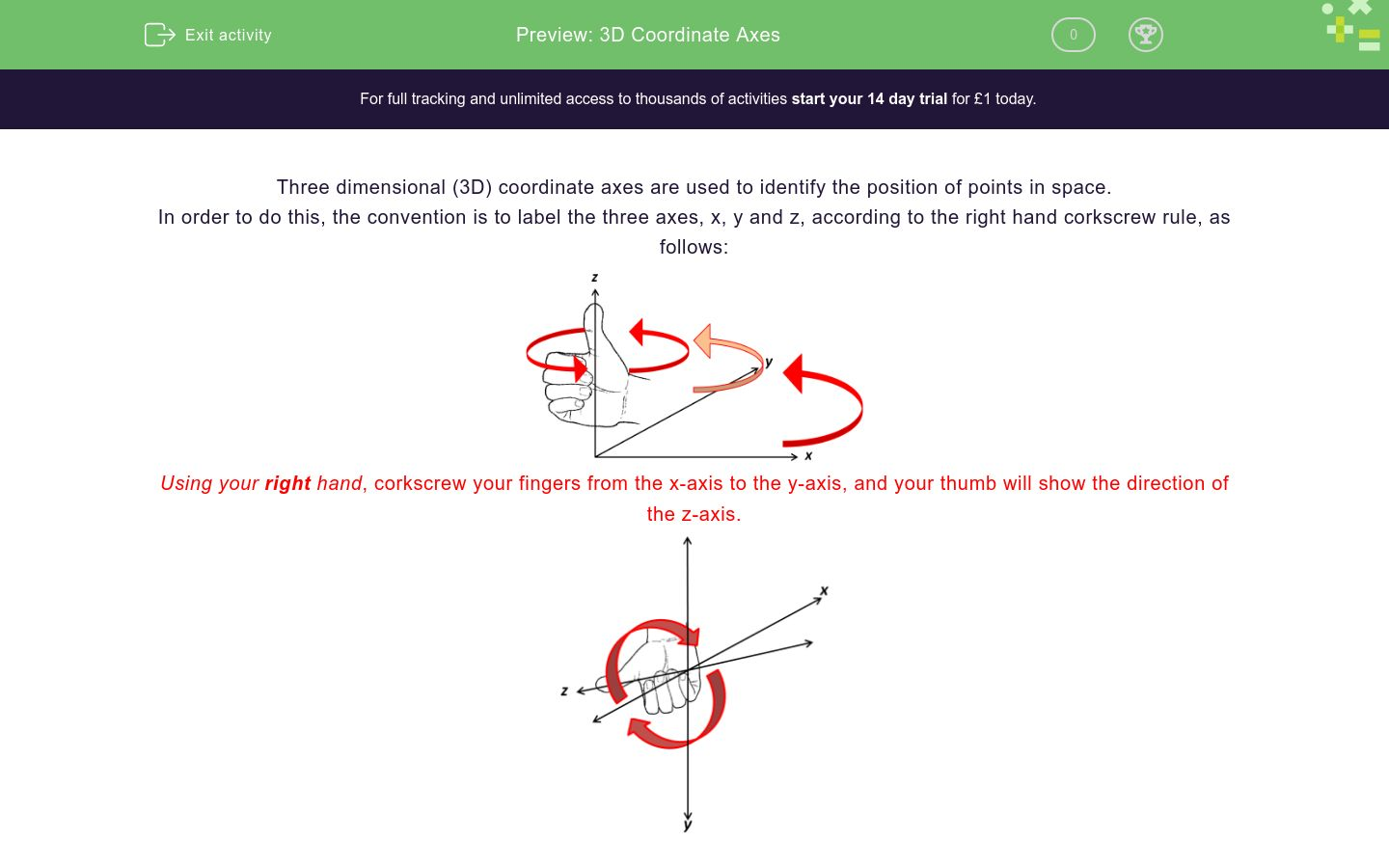# 3D Coordinate Axes

In this worksheet, student label 3D coordinate axes conventionally using the right hand corkscrew rule.Key stage:  KS 4

Curriculum topic:  Geometry and Measures

Curriculum subtopic:  Construct and Interpret Plans and Elevations of 3D Shapes

Difficulty level:### QUESTION 1 of 10

Three dimensional (3D) coordinate axes are used to identify the position of points in space.

In order to do this, the convention is to label the three axes, x, y and z, according to the right hand corkscrew rule, as follows:Using your right hand, corkscrew your fingers from the x-axis to the y-axis, and your thumb will show the direction of the z-axis.In the following set of 3D axes, determine which is the z-axis, given that:

the x-axis is labelled a

the y-axis is labelled ba

b

c

d

e

f

In the following set of 3D axes, determine which is the z-axis, given that:

the x-axis is labelled b

the y-axis is labelled ca

b

c

d

e

f

In the following set of 3D axes, determine which is the z-axis, given that:

the x-axis is labelled b

the y-axis is labelled aa

b

c

d

e

f

In the following set of 3D axes, determine which is the z-axis, given that:

the x-axis is labelled b

the y-axis is labelled ea

b

c

d

e

f

In the following set of 3D axes, determine which is the z-axis, given that:

the x-axis is labelled d

the y-axis is labelled ea

b

c

d

e

f

In the following set of 3D axes, determine which is the z-axis, given that:

the x-axis is labelled d

the y-axis is labelled aa

b

c

d

e

f

In the following set of 3D axes, determine which is the z-axis, given that:

the x-axis is labelled c

the y-axis is labelled ea

b

c

d

e

f

In the following set of 3D axes, determine which is the z-axis, given that:

the x-axis is labelled d

the y-axis is labelled fa

b

c

d

e

f

In the following set of 3D axes, determine which is the z-axis, given that:

the x-axis is labelled d

the y-axis is labelled fa

b

c

d

e

f

In the following set of 3D axes, determine which is the z-axis, given that:

the x-axis is labelled a

the y-axis is labelled ba

b

c

d

e

f

• Question 1

In the following set of 3D axes, determine which is the z-axis, given that:

the x-axis is labelled a

the y-axis is labelled be
EDDIE SAYS
Using the right hand corkscrew rule, the thumb will point along the axis labelled e.
• Question 2

In the following set of 3D axes, determine which is the z-axis, given that:

the x-axis is labelled b

the y-axis is labelled ce
EDDIE SAYS
Using the right hand corkscrew rule, the thumb will point along the axis labelled e.
• Question 3

In the following set of 3D axes, determine which is the z-axis, given that:

the x-axis is labelled b

the y-axis is labelled af
EDDIE SAYS
Using the right hand corkscrew rule, the thumb will point along the axis labelled f.
• Question 4

In the following set of 3D axes, determine which is the z-axis, given that:

the x-axis is labelled b

the y-axis is labelled ea
EDDIE SAYS
Using the right hand corkscrew rule, the thumb will point along the axis labelled a.
• Question 5

In the following set of 3D axes, determine which is the z-axis, given that:

the x-axis is labelled d

the y-axis is labelled ec
EDDIE SAYS
Using the right hand corkscrew rule, the thumb will point along the axis labelled c.
• Question 6

In the following set of 3D axes, determine which is the z-axis, given that:

the x-axis is labelled d

the y-axis is labelled ae
EDDIE SAYS
Using the right hand corkscrew rule, the thumb will point along the axis labelled e.
• Question 7

In the following set of 3D axes, determine which is the z-axis, given that:

the x-axis is labelled c

the y-axis is labelled eb
EDDIE SAYS
Using the right hand corkscrew rule, the thumb will point along the axis labelled b.
• Question 8

In the following set of 3D axes, determine which is the z-axis, given that:

the x-axis is labelled d

the y-axis is labelled fa
EDDIE SAYS
Using the right hand corkscrew rule, the thumb will point along the axis labelled a.
• Question 9

In the following set of 3D axes, determine which is the z-axis, given that:

the x-axis is labelled d

the y-axis is labelled fc
EDDIE SAYS
Using the right hand corkscrew rule, the thumb will point along the axis labelled c.
• Question 10

In the following set of 3D axes, determine which is the z-axis, given that:

the x-axis is labelled a

the y-axis is labelled b